### Learn Multiplication Tables 2 to 20

Tables 2 to 20 are reviewed in this article: Learning the multiplication table is very important in school since it makes tackling large multiplication, division, fraction, and algebra questions easier and faster. Check out the list of multiplication tables from 2 to 20 in the section below and memorise them. When you recall arithmetic tables 2 to 20 by memory as a school student, it raises your confidence in doing long calculations in a shorter time period and increases your interest in mathematics. Scroll down the page to learn the math tables 2 to 20 in an easy and effective manner using the building block framework. Check out some table-picking strategies and advice as well.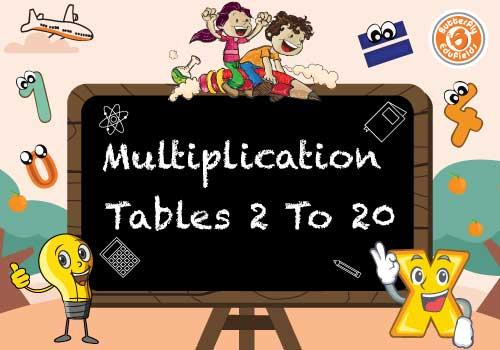### Classification of Tables 2 to 20

Tables 1 to 10 and Tables 11 to 20 have been divided into two sections based on their difficulty: Tables 1 to 10 and Tables 11 to 20. Choose the chart that corresponds to your class and begin memorising tables, as this is the most crucial component for any student in any grade level.

### Multiplication Tables 1 to 10

Building blocks memorise tables from 1 to 10 in a sensible and efficient manner. For children in the fifth standard, learning Tables 2 to 10 would be beneficial.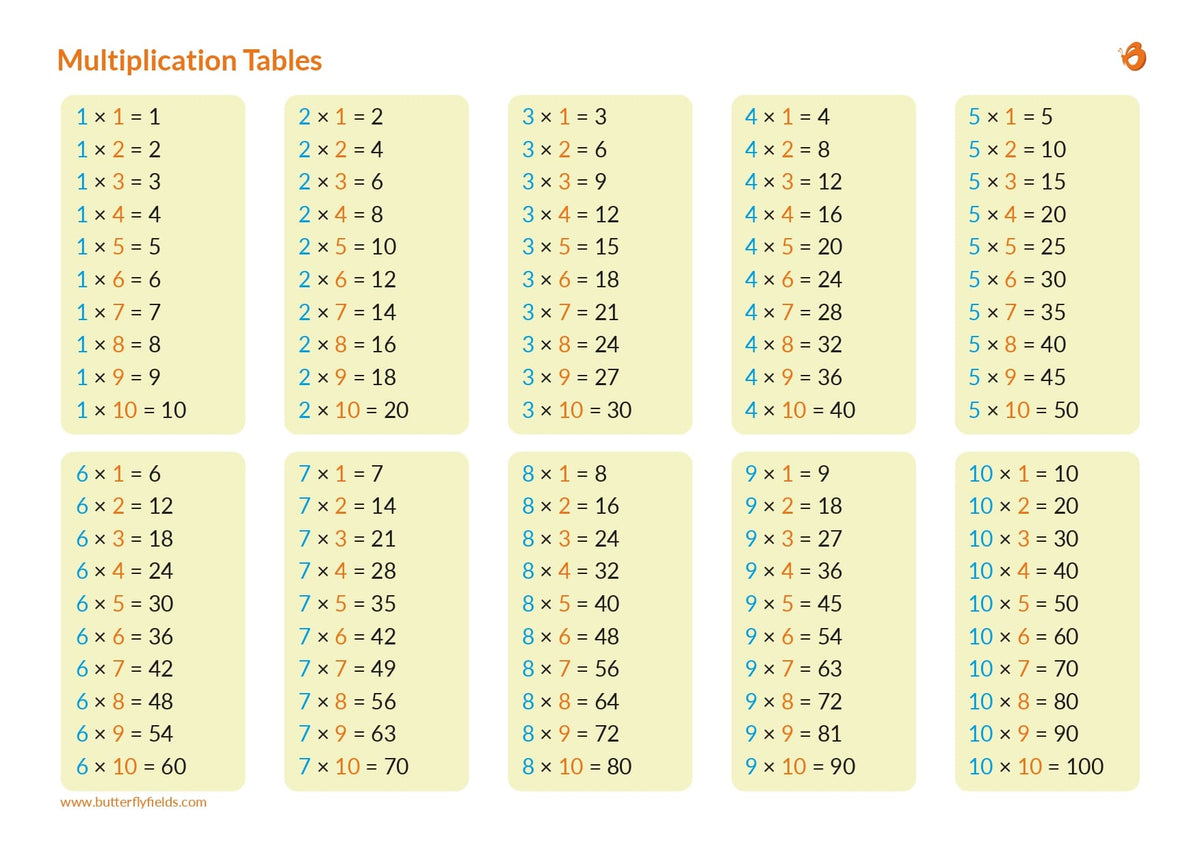### Multiplication Tables 11 to 20

When you get to a higher level, math tables 11 to 20 will help you save time when tackling long and difficult mathematical problems. Tables 11 to 20 can be easily learned using the graphic below.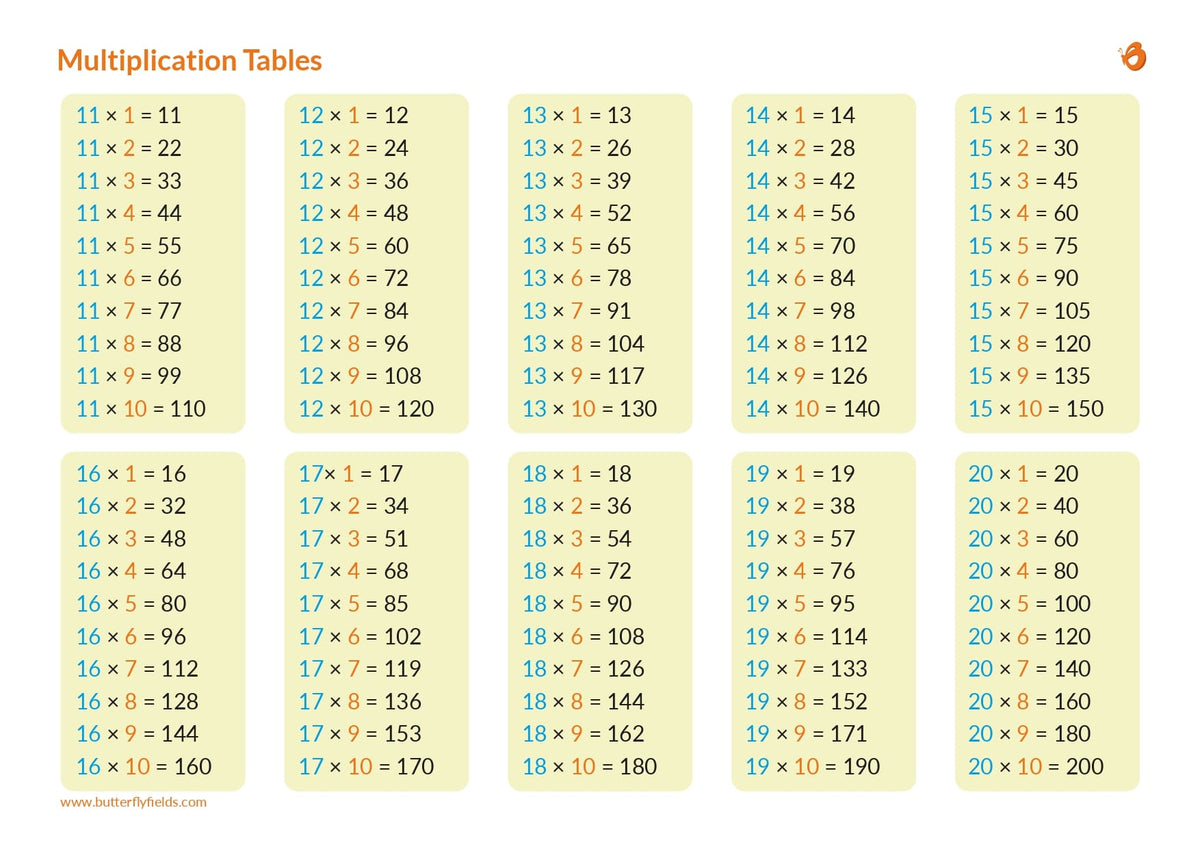### Multiplication Tables 2 to 20

Improve your speed and accuracy in mathematical calculations by learning arithmetic tables 1-20 using the multiplication charts listed below. It's crucial to grasp the notion of arithmetic tables 1 to 20 if you want to avoid making mistakes when doing extensive computations. Learn Tables 1–20 and solve algorithms, HCF-LCM, multiplications, divisions, and other questions in your head in seconds.

### Some Easy Tips to Learn Tables 2 to 20

1. The quickest technique to memorise tables is to vocalise them in a repetition mode or write them down on paper. Let's look at various strategies for learning tables 2 to 20. Remember that every number from 2 to 20 in a multiplication table is a whole number.

2. When a number is multiplied by two in a table of two, it is increased by two times or doubled. For example, 2 times 6 signifies that 6 has been doubled; so, the outcome is 12. 2,4,6,8,10,12,14,16,18,20 could be written as 2,4,6,8,10,12,14,16,18,20.

3. The pattern on the table of 5 is straightforward. The alternate multiplied number has two possible outcomes: 0 and 5. As an example, the table of 5 has the numbers 5,10,15,20,25, ...

4. Table 9 can be remembered by remembering that the unit place digits of the numbers go in decreasing order from 9 to 0 and the ten's place digits of the numbers move in rising order from 0 to 9. 01,18, 27, 36, 45, 54, 63, 72, 81, 90, and so on.

5. Trick of learning Multiplication Table of 13: Adding natural numbers to the 10s digit of the multiples of 3 will be the shortcut to table of 13: (1+0) 3, (2+0)6, (3+0) 9, (4+1) 2, (5+1)5, (6+1) 8, (7+2) 1, (8+2)4, (9+2)7, (10+3) 0 = 13, 26, 39, 52, 65, 78, 91, 104, 117, 130.

6. To memorize the table of 19, write 10 odd numbers in a series at the tens' place, then write the numbers from 0 to 9 in the unit's place starting from the bottom.

### Tables 2 to 5

Table 2Table 3table 4
2 × 1 = 23 × 1 = 34 × 1 = 4
2 × 2 = 43 × 2 = 64 × 2 = 8
2 × 3 = 63 × 3 = 94 × 3 = 12
2 × 4 = 83 × 4 = 124 × 4 = 16
2 × 5 = 103 × 5 = 154 × 5 = 20
2 × 6 = 123 × 6 = 184 × 6 = 24
2 × 7 = 143 × 7 = 214 × 7 = 28
2 × 8 = 163 × 8 = 244 × 8 = 32
2 × 9 = 183 × 9 = 274 × 9 = 36
2 × 10 = 203 × 10 = 304 × 10 = 40

### Tables 6 to 10

Table 6Table 7table 8table 9table 10
6 × 1 = 67 × 1 = 78 × 1 = 89 × 1 = 910 × 1 = 10
6 × 2 = 127 × 2 = 148 × 2 = 169 × 2 = 1810 × 2 = 20
6 × 3 = 187 × 3 = 218 × 3 = 249 × 3 = 2710 × 3 = 30
6 × 4 = 247 × 4 = 288 × 4 = 329 × 4 = 3610 × 4 = 40
6 × 5 = 307 × 5 = 358 × 5 = 409 × 5 = 4510 × 5 = 50
6 × 6 = 367 × 6 = 428 × 6 = 489 × 6 = 5410 × 6 = 60
6 × 7 = 427 × 7 = 498 × 7 = 569 × 7 = 6310 × 7 = 70
2 × 8 = 167 × 8 = 568 × 8 = 649 × 8 = 7210 × 8 = 80
6 × 9 = 547 × 9 = 638 × 9 = 729 × 9 = 8110 × 9 = 90
6 × 10 = 607 × 10 = 708 × 10 = 809 × 10 = 9010 × 10 = 100

### Tables 11 to 15

Table of 11Table 12table 13table 14table 15
11 × 1 = 1112 × 1 = 1213 × 1 = 1314 × 1 = 1415 × 1 = 15
11 × 2 = 2212 × 2 = 2413 × 2 = 2614 × 2 = 2815 × 2 = 30
11 × 3 = 3312 × 3 = 3613 × 3 = 3914 × 3 = 4215 × 3 = 45
11 × 4 = 4412 × 4 = 4813 × 4 = 5214 × 4 = 5615 × 4 = 60
11 × 5 = 5512 × 5 = 6013 × 5 = 6514 × 5 = 7015 × 5 = 75
11 × 6 = 6612 × 6 = 7213 × 6 = 7814 × 6 = 8415 × 6 = 90
11 × 7 = 7712 × 7 = 8413 × 7 = 9114 × 7 = 9815 × 7 = 105
11 × 8 = 8812 × 8 = 9613 × 8 = 10414 × 8 = 11215 × 8 = 120
11 × 9 = 9912 × 9 = 10813 × 9 = 11714 × 9 = 12615 × 9 = 135
11 × 10 = 11012 × 10 = 12013 × 10 = 13014 × 10 = 14015 × 10 = 150

### Tables 16 to 20

Table of 16Table 17table 18table 19table 20
16 × 1 = 1617 × 1 = 1718 × 1 = 1819 × 1 = 1920 × 1 = 20
16 × 2 = 3217 × 2 = 3418 × 2 = 3619 × 2 = 3820 × 2 = 40
16 × 3 = 4817 × 3 = 5118 × 3 = 5419 × 3 = 5720 × 3 = 60
16 × 4 = 6417 × 4 = 6818 × 4 = 7219 × 4 = 7620 × 4 = 80
16 × 5 = 8017 × 5 = 8518 × 5 = 9019 × 5 = 9520 × 5 = 100
16 × 6 = 9617 × 6 = 10218 × 6 = 10819 × 6 = 11420 × 6 = 120
16 × 7 = 11217 × 7 = 11918 × 7 = 12619 × 7 = 13320 × 7 = 140
16 × 8 = 12817 × 8 = 13618 × 8 = 14419 × 8 = 15220 × 8 = 160
16 × 9 = 14417 × 9 = 15318 × 9 = 16219 × 9 = 17120 × 9 = 180
16 × 10 = 16017 × 10 = 17018 × 10 = 18019 × 10 = 19020 × 10 = 200

### Advantages of Learning Tables 2 to 20

Instead of cramming, it is critical for students to learn Math tables 2 to 20. The following are some of the advantages of memorising tables 2 to 20.

1. Allows you to solve more mathematical problems in less time.

2. Increase your self-assurance by knowing the tables from 2 to 20 at your fingertips.

3. Saves time when solving math problems such as arithmetic, long division, fractions, and more.

4. Enhance your enthusiasm for the subject of mathematics, which is critical for success in school.

5. When you memorise tables 2 to 20, you may dispense your skills in class when your teachers ask you to recite them.

6. I hope this post helps you to learn tables from 2 to 20 and increase your self-confidence while handling long calculative problems in less time.

### Shop By Age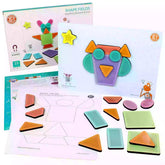1.5+ yrs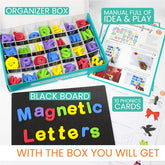4+ yrs6+ yrs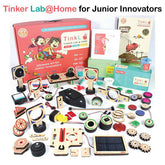8+ yrs10+ yrs13+ yrs

### Our Best Sellers### Wooden ABC & Number Puzzle | 3-5 yrs

Rs. 419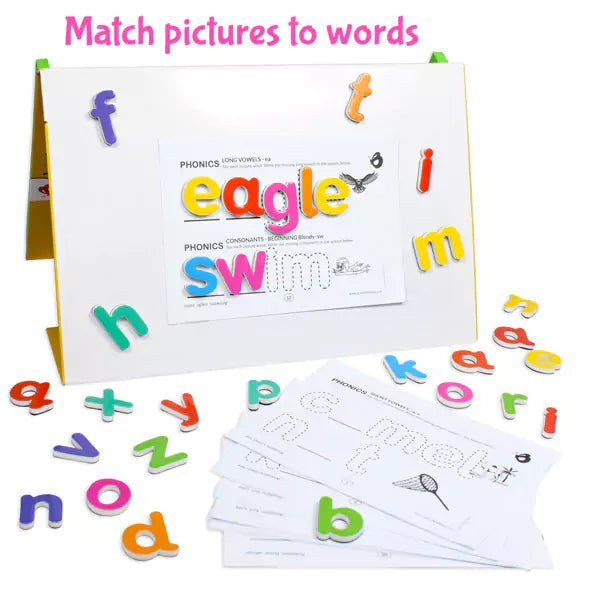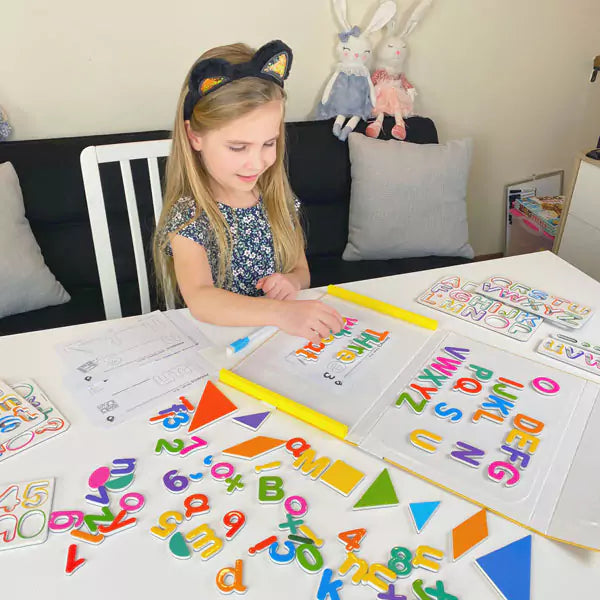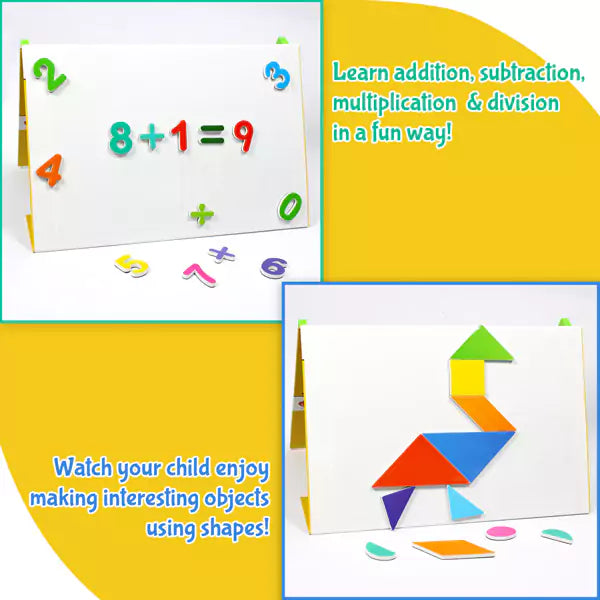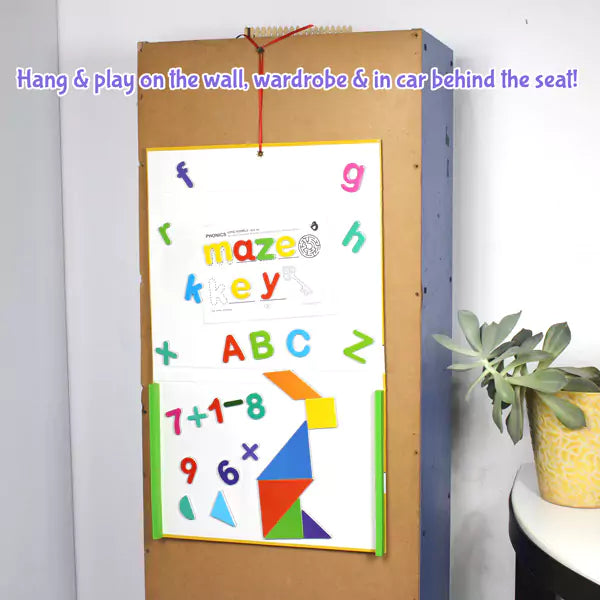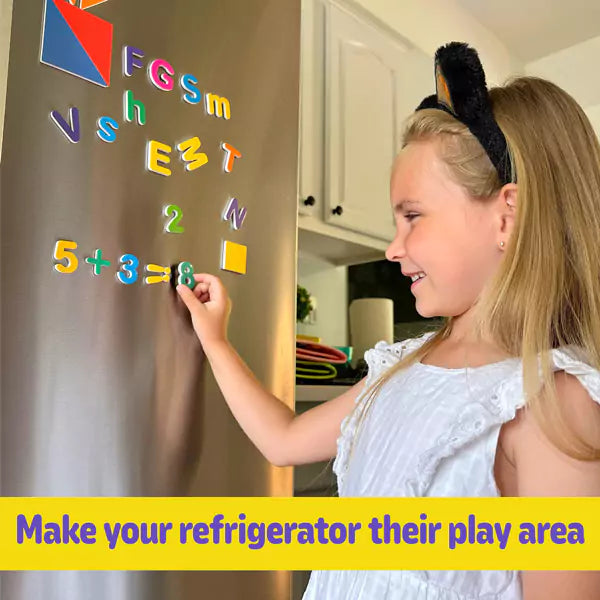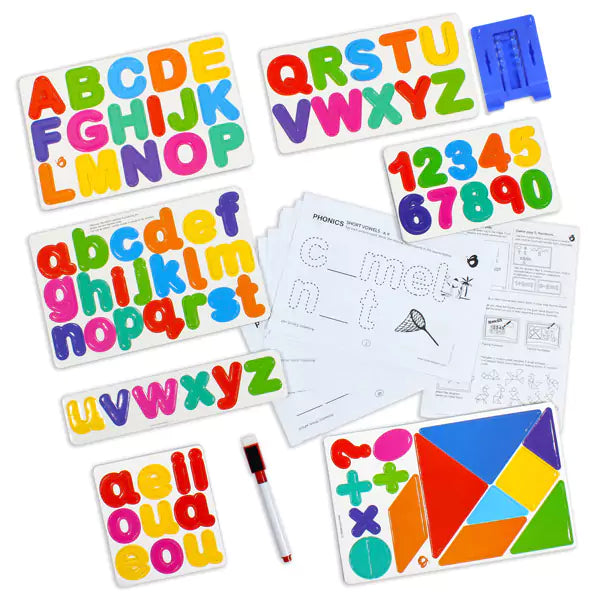### 90pc Magnetic ABC & Writing Board | 3-5 yrs

Rs. 699### ABC & 123 Magnetic Set | 3-5 yrs

Rs. 399### Ultimate Saver Pack | 3-5 yrs

Rs. 1,799
Sunday,Monday,Tuesday,Wednesday,Thursday,Friday,Saturday
January,February,March,April,May,June,July,August,September,October,November,December
Not enough items available. Only [max] left.
Shopping cart